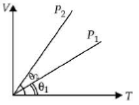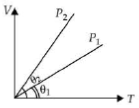Graphs
Question

# In the given (V - T) diagram, what is the relation between pressuresModerate
Solution

## According to ideal gas equationPV = nRTOr $V=\frac{nRT}{P}$For an isobaric process, P = constant and $V\propto T$Therefore, V - T graph is a straight line passing through origin. Slope of this line is inversely proportional to P.In the given figure,Get Instant Solutions